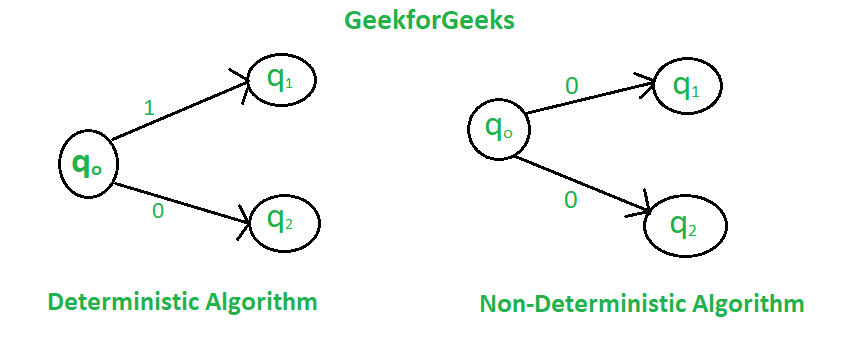Difference between Deterministic and Non-deterministic Algorithms

In deterministic algorithm, for a given particular input, the computer will always produce the same output going through the same states but in case of non-deterministic algorithm, for the same input, the compiler may produce different output in different runs. In fact non-deterministic algorithms can’t solve the problem in polynomial time and can’t determine what is the next step. The non-deterministic algorithms can show different behaviors for the same input on different execution and there is a degree of randomness to it.To implement a non-deterministic algorithm, we have a couple of languages like Prolog but these don’t have standard programming language operators and these operators are not a part of any standard programming languages.

Some of the terms related to the non-deterministic algorithm are defined below:

• choice(X) : chooses any value randomly from the set X.
• failure() : denotes the unsuccessful solution.
• success() : Solution is successful and current thread terminates.

Example :

Problem Statement : Search an element x on A[1:n] where n>=1, on successful search return j if a[j] is equals to x otherwise return 0.

Non-deterministic Algorithm for this problem :

1.j= choice(a, n)
2.if(A[j]==x) then
{
write(j);
success();
}
3.write(0); failure();
Deterministic Algorithm Non-deterministic Algorithm
For a particular input the computer will give always same output. For a particular input the computer will give different output on different execution.
Can solve the problem in polynomial time. Can’t solve the problem in polynomial time.
Can determine the next step of execution. Cannot determine the next step of execution due to more than one path the algorithm can take.

My Personal Notes arrow_drop_upCheck out this Author's contributed articles.

If you like GeeksforGeeks and would like to contribute, you can also write an article using contribute.geeksforgeeks.org or mail your article to contribute@geeksforgeeks.org. See your article appearing on the GeeksforGeeks main page and help other Geeks.

Please Improve this article if you find anything incorrect by clicking on the "Improve Article" button below.